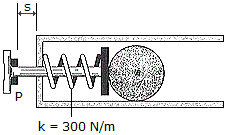# Engineering Mechanics - KOP: Work and Energy - Discussion

### Discussion :: KOP: Work and Energy - General Questions (Q.No.4)

4.The firing mechanism of a pinball machine consists of a plunger P having a mass of 0.25 kg and a spring of stiffness k = 300 N/m. When s = 0, the spring is compressed 50 mm. If the arm is pulled back such that s = 100 mm and released, determine the speed of the 0.3 kg pinball B just before the plunger strikes the stop, i.e., s = 0. Assume all sufaces of contact to be smooth. The ball moves in the horizontal plane. Note that the ball slides without rolling.

 [A]. v = 4.47 m/s [B]. v = 3.30 m/s [C]. v = 2.34 m/s [D]. v = 3.16 m/s

Explanation:

No answer description available for this question.

 Jani Dhruvkumar said: (Aug 3, 2019) (1/2)(300)[(0+50)^2] = (1/2)(300)[(100+50)^2] + (1/2)(.3+.25)(v^2). V = 3.30m/s.